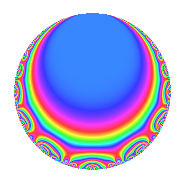# Properties

 Label 2011.1.b.aLevel 2011 Weight 1 Character orbit 2011.b Self dual Yes Analytic conductor 1.004 Analytic rank 0 Dimension 3 Projective image $$D_{7}$$ CM disc. -2011 Inner twists 2

# Related objects

## Newspace parameters

 Level: $$N$$ = $$2011$$ Weight: $$k$$ = $$1$$ Character orbit: $$[\chi]$$ = 2011.b (of order $$2$$ and degree $$1$$)

## Newform invariants

 Self dual: Yes Analytic conductor: $$1.0036197404$$ Analytic rank: $$0$$ Dimension: $$3$$ Coefficient field: $$\Q(\zeta_{14})^+$$ Coefficient ring: $$\Z[a_1, \ldots, a_{5}]$$ Coefficient ring index: $$1$$ Projective image $$D_{7}$$ Projective field Galois closure of 7.1.8132727331.1 Artin image size $$14$$ Artin image $D_7$ Artin field Galois closure of 7.1.8132727331.1

## $q$-expansion

Coefficients of the $$q$$-expansion are expressed in terms of a basis $$1,\beta_1,\beta_2$$ for the coefficient ring described below. We also show the integral $$q$$-expansion of the trace form.

 $$f(q)$$ $$=$$ $$q$$ $$+ q^{4}$$ $$-\beta_{1} q^{5}$$ $$+ q^{9}$$ $$+O(q^{10})$$ $$q$$ $$+ q^{4}$$ $$-\beta_{1} q^{5}$$ $$+ q^{9}$$ $$+ ( -1 + \beta_{1} - \beta_{2} ) q^{13}$$ $$+ q^{16}$$ $$-\beta_{1} q^{20}$$ $$+ \beta_{2} q^{23}$$ $$+ ( 1 + \beta_{2} ) q^{25}$$ $$+ \beta_{2} q^{31}$$ $$+ q^{36}$$ $$+ ( -1 + \beta_{1} - \beta_{2} ) q^{41}$$ $$+ ( -1 + \beta_{1} - \beta_{2} ) q^{43}$$ $$-\beta_{1} q^{45}$$ $$+ q^{49}$$ $$+ ( -1 + \beta_{1} - \beta_{2} ) q^{52}$$ $$+ q^{64}$$ $$+ ( -1 + \beta_{1} ) q^{65}$$ $$+ ( -1 + \beta_{1} - \beta_{2} ) q^{71}$$ $$-\beta_{1} q^{80}$$ $$+ q^{81}$$ $$+ \beta_{2} q^{83}$$ $$+ \beta_{2} q^{89}$$ $$+ \beta_{2} q^{92}$$ $$+O(q^{100})$$ $$\operatorname{Tr}(f)(q)$$ $$=$$ $$3q$$ $$\mathstrut +\mathstrut 3q^{4}$$ $$\mathstrut -\mathstrut q^{5}$$ $$\mathstrut +\mathstrut 3q^{9}$$ $$\mathstrut +\mathstrut O(q^{10})$$ $$3q$$ $$\mathstrut +\mathstrut 3q^{4}$$ $$\mathstrut -\mathstrut q^{5}$$ $$\mathstrut +\mathstrut 3q^{9}$$ $$\mathstrut -\mathstrut q^{13}$$ $$\mathstrut +\mathstrut 3q^{16}$$ $$\mathstrut -\mathstrut q^{20}$$ $$\mathstrut -\mathstrut q^{23}$$ $$\mathstrut +\mathstrut 2q^{25}$$ $$\mathstrut -\mathstrut q^{31}$$ $$\mathstrut +\mathstrut 3q^{36}$$ $$\mathstrut -\mathstrut q^{41}$$ $$\mathstrut -\mathstrut q^{43}$$ $$\mathstrut -\mathstrut q^{45}$$ $$\mathstrut +\mathstrut 3q^{49}$$ $$\mathstrut -\mathstrut q^{52}$$ $$\mathstrut +\mathstrut 3q^{64}$$ $$\mathstrut -\mathstrut 2q^{65}$$ $$\mathstrut -\mathstrut q^{71}$$ $$\mathstrut -\mathstrut q^{80}$$ $$\mathstrut +\mathstrut 3q^{81}$$ $$\mathstrut -\mathstrut q^{83}$$ $$\mathstrut -\mathstrut q^{89}$$ $$\mathstrut -\mathstrut q^{92}$$ $$\mathstrut +\mathstrut O(q^{100})$$

Basis of coefficient ring in terms of $$\nu = \zeta_{14} + \zeta_{14}^{-1}$$:

 $$\beta_{0}$$ $$=$$ $$1$$ $$\beta_{1}$$ $$=$$ $$\nu$$ $$\beta_{2}$$ $$=$$ $$\nu^{2} - 2$$
 $$1$$ $$=$$ $$\beta_0$$ $$\nu$$ $$=$$ $$\beta_{1}$$ $$\nu^{2}$$ $$=$$ $$\beta_{2}\mathstrut +\mathstrut$$ $$2$$

## Character Values

We give the values of $$\chi$$ on generators for $$\left(\mathbb{Z}/2011\mathbb{Z}\right)^\times$$.

 $$n$$ $$3$$ $$\chi(n)$$ $$-1$$

## Embeddings

For each embedding $$\iota_m$$ of the coefficient field, the values $$\iota_m(a_n)$$ are shown below.

For more information on an embedded modular form you can click on its label.

Label $$\iota_m(\nu)$$ $$a_{2}$$ $$a_{3}$$ $$a_{4}$$ $$a_{5}$$ $$a_{6}$$ $$a_{7}$$ $$a_{8}$$ $$a_{9}$$ $$a_{10}$$
2010.1
 1.80194 0.445042 −1.24698
0 0 1.00000 −1.80194 0 0 0 1.00000 0
2010.2 0 0 1.00000 −0.445042 0 0 0 1.00000 0
2010.3 0 0 1.00000 1.24698 0 0 0 1.00000 0
 $$n$$: e.g. 2-40 or 990-1000 Significant digits: Format: Complex embeddings Normalized embeddings Satake parameters Satake angles

## Inner twists

Char. orbit Parity Mult. Self Twist Proved
1.a Even 1 trivial yes
2011.b Odd 1 CM by $$\Q(\sqrt{-2011})$$ yes

## Hecke kernels

There are no other newforms in $$S_{1}^{\mathrm{new}}(2011, [\chi])$$.